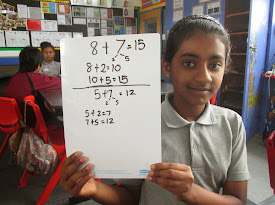## Friday, 13 March 2015

### Saruja- Math strategyOn Monday my group did math with Mrs Anderson. We learnt about how to solve addition question by first making ten and the adding what's left over.  Wed did this for example so we will know how to do this activity. This math is good because you breaking the number into ten. For example 8+7=?  so you break the seven . Which it was 2 and 5 and you plus 8 and 2 and the answer is 10 after that you plus 10 and 5 which is make 15. You got the 8+7=15. This math skills help me to do it well.I think this is a easy way to do your addition at school or at home.